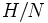# Quotient-transitive subgroup property

This article defines a subgroup metaproperty: a property that can be evaluated to true/false for any subgroup property
View a complete list of subgroup metaproperties
View subgroup properties satisfying this metaproperty| View subgroup properties dissatisfying this metaproperty
VIEW RELATED: subgroup metaproperty satisfactions| subgroup metaproperty dissatisfactions

BEWARE! This term is nonstandard and is being used locally within the wiki. [SHOW MORE]

## Definition

### Definition with symbols

Let$p$ be a subgroup property. Then,$p$ is said to be quotient-transitive if whenever$H$ is a subgroup of$G$ such that there is a normal subgroup$N$ of$G$ contained in$H$ then the first of these implies the second:

•$N$ satisfies$p$ in$G$ and$H/N$ satisfies$p$ in$G/N$.
•$H$ satisfies$p$ in$G$.

### In terms of the quotient-composition operator

A subgroup property$p$ is termed quotient-transitive if it is transitive with respect to the quotient-composition operator.

## Property theory

### Remedies for lack of quotient-transitivity

There are three general ways to pass from a subgroup property to a quotient-transitive variation. These are analogous to the three ways to pass to an analogous transitive subgroup property.

## Naturally arising quotient-transitive subgroup properties

### Because of invariance

Any invariance property with respect to a quotient-hereditary function property is quotient-transitive. Examples are: